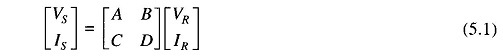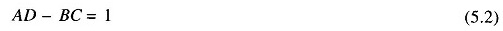## Introduction to Power Transmission Line:

Introduction to Power Transmission Line deals primarily with the characteristics and performance of transmission lines. A problem of major importance in power systems is the flow of load over transmission lines such that the voltage at various nodes is maintained within specified limits.

Transmission lines are normally operated with a balanced three-phase load; the analysis can therefore proceed on a per phase basis. A transmission line on a per phase basis can be regarded as a two-port network, wherein the sending-end voltage VS and current IS are related to the receiving-end voltage VR and current IR through ABCD constants asAlso the following identity holds for ABCD constants:These constants can be determined easily for short and medium-length lines by suitable approximations lumping the line impedance and shunt admittance. For long lines exact analysis has to be carried out by considering the distribution of resistance, inductance and capacitance parameters and the ABCD constants of the line are determined therefrom. Equations for power flow on a line and receiving- and sending-end circle diagrams will also be developed in this chapter so that various types of end conditions can be handled.

The following nomenclature has been adopted in the discussion of Introduction to Power Transmission Line:

• z = series impedance/unit length/phase
• y = shunt admittance/unit length/phase to neutral
• r = resistance/unit length/phase
• L = inductance/unit length/phase
• C = capacitance/unit length/phase to neutral
• l = transmission line length
• Z = zl = total series impedance/phase
• Y= yl = total shunt admittance/phase to neutral

Noted:

Subscript S stands for a sending end quantity and subscript R stands for a receiving end quantity.

Scroll to Top